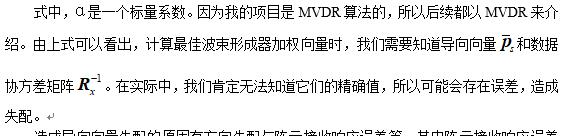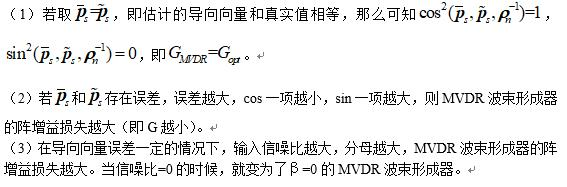2019-10-16  500 views 1

# 《优化阵列信号处理》学习笔记（第四章）

## 第四章：波束稳健性分析

### 4.1 最佳波束形成器稳健性影响因素

$${{w}{opt}}=\alpha R{x}^{-1}{{\bar{p}}_{s}}$$### 4.2 导向向量失配对波束性能的影响

$${{G}{MVDR}}({{\bar{p}}{s}}:{{\tilde{p}}{s}})=\frac{{{\left| \bar{p}{s}^{H}R_{x}^{-1}{{{\tilde{p}}}{s}} \right|}^{2}}}{\bar{p}{s}^{H}R_{x}^{-1}{{\rho }{n}}R{x}^{-1}{{{\bar{p}}}_{s}}}$$

$${{G}{MVDR}}=\frac{\bar{p}{s}^{H}\rho {n}^{-1}{{{\tilde{p}}}{s}}{{\cos }^{2}}({{{\bar{p}}}{s}},{{{\tilde{p}}}{s}},\rho {n}^{-1})}{1+{2(\sigma _{s}^{2}/\sigma _{n}^{2})\tilde{p}{s}^{H}\rho {n}^{-1}{{{\tilde{p}}}{s}}+{{[(\sigma {s}^{2}/\sigma _{n}^{2})\tilde{p}{s}^{H}\rho {n}^{-1}{{{\tilde{p}}}{s}}]}^{2}}}{{\sin }^{2}}({{{\bar{p}}}{s}},{{{\tilde{p}}}{s}},\rho _{n}^{-1})}$$### 4.3 协方差矩阵失配对波束性能的影响

4.3.1 样本协方差矩阵求逆波束形成

$${{w}{MVDR}}=\alpha {{R}^{-1}}{{\bar{p}}{s}}$$

$$\alpha \text{=}{{(\bar{p}{s}^{H}{{R}^{-1}}{{\bar{p}}{s}})}^{-1}}$$

$$\hat{R}\text{=}\frac{1}{N}\sum\limits_{n=1}^{N}{[x(n){{x}^{H}}(n)]}$$

$${{w}{MVDR}}=\alpha {{\hat{R}}^{-1}}{{\bar{p}}{s}}$$

$$O({{M}^{3}})$$

4.3.2 样本协方差矩阵求逆法波束性能

$${{\rho }{0}}\text{=}{{\left. \frac{SINR({{{\hat{w}}}{SMI}})}{SIN{{R}{opt}}} \right|}{\beta =0}}$$

$$E({{\rho }_{0}})=\frac{N-M+2}{N+1}$$

$$N\ge 2M-3\approx 2M$$

$$E(SLL)\text{=}\frac{1}{N+1}$$

$$N\ge SIN{{R}_{opt}}\centerdot (M-1)\gg M$$

pdf版本下载链接: https://pan.baidu.com/s/1kR8lqyWiDmygWE27fwVwSQ## 来自外部的引用： 1 条

• 个人学习笔记整理 – 小奥の部落格